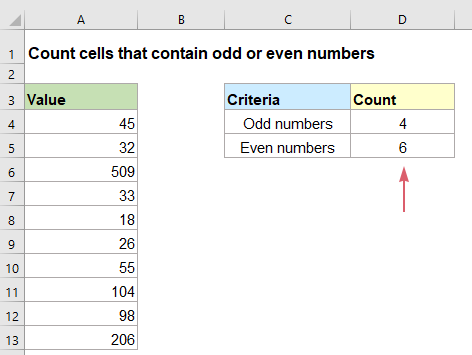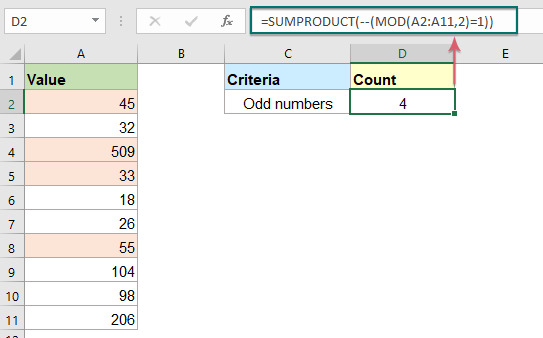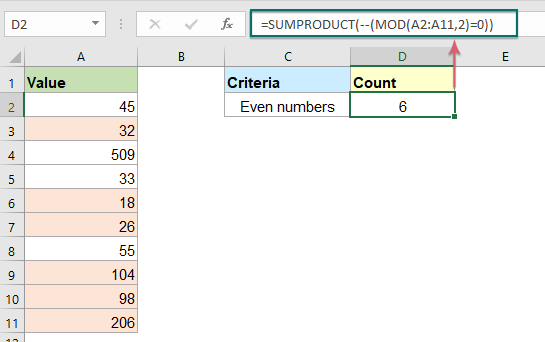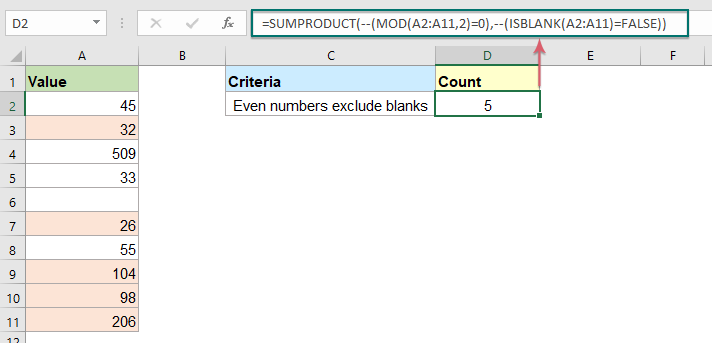## Count number of cells that contain odd or even numbers in excel

As we all known, odd numbers have a remainder of 1 when divided by 2, and the even numbers have a remainder of 0 when divided by 2. This tutorial, I will talk about how to get the number of cells that contain odd or even numbers in Excel.#### Count number of cells that contain odd numbers

To get the number of cells that contain odd numbers only, the combination of SUMPRODUCT and MOD function can help you, the generic syntax is:

=SUMPRODUCT(--(MOD(range,2)=1))
• range: This is the range of cells which you want to count all odd numbers from.

Please enter or copy the below formula into a blank cell, and then press Enter key to get the result, see screenshot:

=SUMPRODUCT(--(MOD(A2:A11,2)=1))##### Explanation of the formula:
• MOD(A2:A11,2): This MOD function returns a remainder after division. In this case, the divisor is 2, so MOD will return a remainder of 1 for odd numbers, and a remainder of 0 for even numbers. So, you will get the result as this: {1;0;1;1;0;0;1;0;0;0}.
• MOD(A2:A11,2)=1: This part of the formula will create an array of Boolean values where TRUE indicates the number is odd and FALSE indicates the number is not odd. This will get the result as this: {TRUE;FALSE;TRUE;TRUE;FALSE;FALSE;TRUE;FALSE;FALSE;FALSE}.
• --(MOD(A2:A11,2)=1): The double negative signs will convert the TRUE value to 1, and FALSE value to 0 as this: {1;0;1;1;0;0;1;0;0;0}.
• SUMPRODUCT(--(MOD(A2:A11,2)=1))=SUMPRODUCT({1;0;1;1;0;0;1;0;0;0}): At last, this SUMPRODUCT function is used to sum the numbers in the array and returns the result: 4.

#### Count number of cells that contain even numbers

If you want to count the number of cells that contain even numbers, the generic syntax is:

=SUMPRODUCT(--(MOD(range,2)=0))
• range: This is the range of cells which you want to count all even numbers from.

Please enter or copy the below formula into a blank cell, and then press Enter key to get the number of all even numbers, see screenshot:

=SUMPRODUCT(--(MOD(A2:A11,2)=0))##### Explanation of the formula:
• MOD(A2:A11,2): This MOD function returns a remainder after division. In this case, the divisor is 2, so MOD will return a remainder of 1 for odd numbers, and a remainder of 0 for even numbers. So, you will get the result as this: {1;0;1;1;0;0;1;0;0;0}.
• MOD(A2:A11,2)=0: This part of the formula will create an array of Boolean values where TRUE indicates the number is even and FALSE indicates the number is not even. This will get the result as this: {FALSE;TRUE;FALSE;FALSE;TRUE;TRUE;FALSE;TRUE;TRUE;TRUE}.
• --(MOD(A2:A11,2)=0):The double negative signs will convert the TRUE value to 1, and FALSE value to 0 as this: {0;1;0;0;1;1;0;1;1;1}.
• SUMPRODUCT(--(MOD(A2:A11,2)=1))=SUMPRODUCT({0;1;0;0;1;1;0;1;1;1}): At last, this SUMPRODUCT function is used to sum the numbers in the array and returns the result: 6.

Tips: The above formula treats blank cells as even numbers as well, if you want the blanks to be excluded, please apply this formula:#### Relative function used:

• SUMPRODUCT:
• The SUMPRODUCT function can be used to multiply two or more columns or arrays together, and then get the sum of products.
• MOD:
• The MOD function returns the remainder of two numbers after division.

#### More articles:

• Count Number Of Cells That Contain Specific Text In Excel
• Supposing, you have a list of text strings, and may want to find the number of cells that contain certain text as part of their contents. In this case, you can use the wildcard characters (*) that represents any texts or characters in your criteria when applying the COUNTIF function. This article, I will introduce how to use formulas for dealing with this job in Excel.
• Count number of cells not equal to many values in Excel
• In Excel, you may easily get the number of cells not equal to a specific value by using the COUNTIF function, but have you ever tried to count the cells which are not equal to many values? For example, I want to get the total number of the products in column A but exclude the specific items in C4:C6 as below screenshot shown. This article, I will introduce some formulas for solving this job in Excel.
• Count Number Of Cells Contain Numeric Or Non-Numeric Values
• If you have a range of data which contains both numeric and non- numeric values, and now, you may want to count the number of numeric or non-numeric cells as below screenshot shown. This article, I will talk about some formulas for solving this task in Excel.

### The Best Office Productivity Tools

#### Kutools for Excel - Helps You To Stand Out From Crowd

 Popular Features: Find/Highlight/Identify Duplicates  |  Delete Blank Rows  |  Combine Columns or Cells without Losing Data  |  Round without Formula ... Super VLookup: Multiple Criteria  |  Multiple Value   |  Across Multi-Sheets  |  Fuzzy Lookup... Adv. Drop-down List: Easy Drop Down List  |  Dependent Drop Down List  |  Multi-select Drop Down List... Column Manager: Add a Specific Number of Columns  |  Move Columns  |  Unhide Columns  |  Compare Columns to Select Same & Different Cells ... Featured Features: Grid Focus  |  Design View  |  Big Formula Bar  |  Workbook & Sheet Manager  |  Resource Library (Auto Text)  |  Date Picker  |  Combine Worksheets  |  Encrypt/Decrypt Cells  |  Send Emails by List  |  Super Filter  |  Special Filter (filter bold/italic/strikethrough...) ... Top 15 Toolset:  12 Text Tools (Add Text, Remove Characters ...)  |  50+ Chart Types (Gantt Chart ...)  |  40+ Practical Formulas (Calculate age based on birthday ...)  |  19 Insertion Tools (Insert QR Code, Insert Picture from Path ...)  |  12 Conversion Tools (Numbers to Words, Currency Conversion ...)  |  7 Merge & Split Tools (Advanced Combine Rows, Split Cells ...)  |  Many More...

Kutools for Excel Boasts Over 300 Features, Ensuring That What You Need is Just A Click Away...

Supports Office/Excel 2007-2021 and 365   |   Available in 44 Languages   |   Full-Featured 30-Day Free Trial.#### Office Tab - Enable Tabbed Reading and Editing in Microsoft Office (include Excel)

• One second to switch between dozens of open documents!
• Reduce hundreds of mouse clicks for you every day, say goodbye to mouse hand.
• Increases your productivity by 50% when viewing and editing multiple documents.
• Brings Efficient Tabs to Office (include Excel), Just Like Chrome, Firefox, And New Internet Explorer.